In :
import pprint
import numpy as np
from Crypto.Random.random import getrandbits


# Prerequisites¶

• Block ciphers design
In :
def get_bit(x, i, n):
'''
n = 5
x = 0 1 0 1 0
i = 0 1 2 3 4
'''
return (x >> (n - 1 - i)) & 1

def inverse_sbox(S):
"""
Input:
S: {list} -- sbox
Return
{list} -- the inverse of the sbox
"""
S_ = [-1] * len(S)
for i, entry in enumerate(S):
S_[entry] = i
return S_



# Theory¶

• $M$ is a message
• $C$ is a ciphertext
• $K$ is a key

Sometimes we will denote

• $X$ - Sbox input
• $Y$ - Sbox output

Otherwise we will follow the notation from the drawings

Idea

• Find an approximate equation of the whole chipher - Link the bits $M_i, C_i$ and $K_i$ using a xor equation (linear equation)
• We want to look after a propriety that "degrades" over the cipher
• We want to take a subset of message bits (masked) and a subset of ciphertext bits(masked) and try to find a probabilistic linear relation between them
• Since SBoxes are the only non-linear component we want to look at those
• We want to find as much information about the key in form of equations

We define the masks as follows:

if $X = (X_0, X_1, X_2, X_3)$ a mask $\alpha = 1001$ selects the bits $X_0$ and $X_3$.

• We can do this with the and operation.
• Moreover since we want a linear relationship we care about the xor between the selected bits so we will encompass both both in the same notation.

We will denote it with $\alpha \cdot X$ (think of it like a dot product)

• For our example $$X_0 \oplus X_3 = \bigoplus_{i=0}^n(\alpha_i X_i) = \alpha \cdot X$$
In :
def bit_parity(x):
'''
Xor of all bits
'''
t = 0
while(x != 0):
t  = t ^ (x & 1)
x >>= 1
return t

# We calculate the parity because it's a xor of all bits
return bit_parity(t)


Now if we want to link the specific input bits to some specific output we use 2 masks ($\alpha$ for the input and $\beta$ for the output ): $$\alpha \cdot X = \beta \cdot S(X) = \beta \cdot Y$$

• where $S$ = our sbox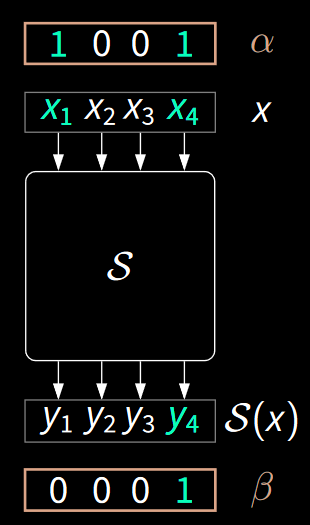## Approximating non-linear functions by linear functions¶

What is a linear function?

$x_n + ... + x_0 + a = y_m + ... + y_0 + b \bmod 2$
We consider linear functions: 0, the input bits alone or any affine transformation between them

Reminder: $\oplus = + \bmod 2$

Example: Consider the and gate

x y xy
0 0 0
0 1 0
1 0 0
1 1 1

We have the following linear functions: $0, x, y, x\oplus y$ and $x\oplus y \oplus 1$

How do we evaluate good approximations? -- We will use different metrics

• Matches = How many outputs match to the input?
• $s = |\{x \in \mathbb{F}_2^n \ : \ \alpha \cdot X = \beta \cdot S(X)\}|$
• Ex: $x$ mathces the and function $3$ times. $x\oplus y$ matches once. (Write the tables)
• Probability $p = P_X[\alpha \cdot X = \beta \cdot S(X) = \dfrac s {2^b}$
• Bias $\epsilon = p - \dfrac 1 2$

• Correlation $cor = 2 \cdot \epsilon$

Interpretaion of the bias

• How different is from random? Random is right $\dfrac 1 2$ times so we subtract it
• $\epsilon = 0$ -- as good as random
• $\epsilon > 0$ -- good appr
• $\epsilon < 0$ -- bad appr but we can transform it into a good one if we xor by 1
In :
# Consider all possible masks and check the results
def count_matches(alpha, beta, sbox, l):
"""
Counts mathches of input output given 2 masks
Input
sbox: {list} -- sbox
l: {int} -- sbox intput size in bits
Returns
{int} -- number of matches
"""
s = 0
for x in range(1<<l):
if dot(x, alpha) == dot(sbox[x], beta):
s+=1
return s

def linear_approximation_table(sbox, l):
"""
Computes the linear approximation table
Input
sbox: {list} -- sbox
l: {int} -- sbox intput size in bits
Returns
{list of lists} -- table
"""
res = []
for alpha in range(1<<l):
row = []
for beta in range(1<<l):
t = count_matches(alpha, beta, sbox, l) - 1/2 * (1<<l) # 1<<l is a normalization factor
row.append(t)
res.append(row)
return res


In :
# An sbox
S = [12, 5, 6, 11, 9, 0, 10, 13, 3, 14, 15, 8, 4, 7, 1, 2]

In :
lat = linear_approximation_table(S, 4)

# Prettier print
print(np.matrix(lat))

[[ 8.  0.  0.  0.  0.  0.  0.  0.  0.  0.  0.  0.  0.  0.  0.  0.]
[ 0.  0.  0.  0.  0. -4.  0. -4.  0.  0.  0.  0.  0. -4.  0.  4.]
[ 0.  0.  2.  2. -2. -2.  0.  0.  2. -2.  0.  4.  0.  4. -2.  2.]
[ 0.  0.  2.  2.  2. -2. -4.  0. -2.  2. -4.  0.  0.  0. -2. -2.]
[ 0.  0. -2.  2. -2. -2.  0.  4. -2. -2.  0. -4.  0.  0. -2.  2.]
[ 0.  0. -2.  2. -2.  2.  0.  0.  2.  2. -4.  0.  4.  0.  2.  2.]
[ 0.  0.  0. -4.  0.  0. -4.  0.  0. -4.  0.  0.  4.  0.  0.  0.]
[ 0.  0.  0.  4.  4.  0.  0.  0.  0. -4.  0.  0.  0.  0.  4.  0.]
[ 0.  0.  2. -2.  0.  0. -2.  2. -2.  2.  0.  0. -2.  2.  4.  4.]
[ 0.  4. -2. -2.  0.  0.  2. -2. -2. -2. -4.  0. -2.  2.  0.  0.]
[ 0.  0.  4.  0.  2.  2.  2. -2.  0.  0.  0. -4.  2.  2. -2.  2.]
[ 0. -4.  0.  0. -2. -2.  2. -2. -4.  0.  0.  0.  2.  2.  2. -2.]
[ 0.  0.  0.  0. -2. -2. -2. -2.  4.  0.  0. -4. -2.  2.  2. -2.]
[ 0.  4.  4.  0. -2. -2.  2.  2.  0.  0.  0.  0.  2. -2.  2. -2.]
[ 0.  0.  2.  2. -4.  4. -2. -2. -2. -2.  0.  0. -2. -2.  0.  0.]
[ 0.  4. -2.  2.  0.  0. -2. -2. -2.  2.  4.  0.  2.  2.  0.  0.]]

In :
# Let's look at another Sbox (Block cipher companion)
S = [0xf, 0xe, 0xb, 0xc, 0x6, 0xd, 0x7, 0x8, 0x0, 0x3, 0x9, 0xa, 0x4, 0x2, 0x1, 0x5]
lat = linear_approximation_table(S, 4)
print(np.matrix(lat))

[[ 8.  0.  0.  0.  0.  0.  0.  0.  0.  0.  0.  0.  0.  0.  0.  0.]
[ 0. -2.  0.  2.  0. -2.  4. -2.  2.  4.  2.  0. -2.  0.  2.  0.]
[ 0.  2. -2.  0. -2.  0.  0.  2.  2.  4.  0.  2.  4. -2. -2.  0.]
[ 0.  4.  2.  2. -2.  2.  0.  0.  0.  0.  2. -2. -2. -2.  0.  4.]
[ 0.  0. -2.  2.  2. -2.  0.  0. -4.  0.  2.  2.  2.  2.  0.  4.]
[ 0. -2.  2.  0.  2.  4.  0.  2. -2.  4.  0. -2.  0.  2. -2.  0.]
[ 0. -2.  0.  2.  0.  2.  4.  2.  2. -4.  2.  0.  2.  0. -2.  0.]
[ 0.  0.  0.  0.  0.  4.  0. -4.  0.  0.  0.  0.  4.  0.  4.  0.]
[ 0.  0. -2.  2. -4.  0.  2.  2. -4.  0. -2. -2.  0.  0.  2. -2.]
[ 0. -2. -6.  0.  0.  2. -2.  0.  2.  0.  0. -2. -2.  0.  0.  2.]
[ 0. -2.  0. -6. -2.  0.  2.  0. -2.  0.  2.  0.  0. -2.  0.  2.]
[ 0.  0.  0.  0.  2. -2.  2. -2.  0.  0. -4. -4.  2. -2. -2.  2.]
[ 0.  0.  0.  0. -2. -2. -2. -2.  0.  0.  4. -4.  2.  2. -2. -2.]
[ 0. -2.  0.  2.  2.  0. -2.  0. -2.  0.  2.  0.  0. -6.  0. -2.]
[ 0.  2. -2.  0.  0.  2.  2. -4. -2.  0.  0.  2. -2.  0. -4. -2.]
[ 0. -4.  2.  2. -4.  0. -2. -2.  0.  0. -2.  2.  0.  0. -2.  2.]]


Adding the key is simple. $$\alpha X \cdot \oplus \kappa \cdot K = \beta \cdot Y$$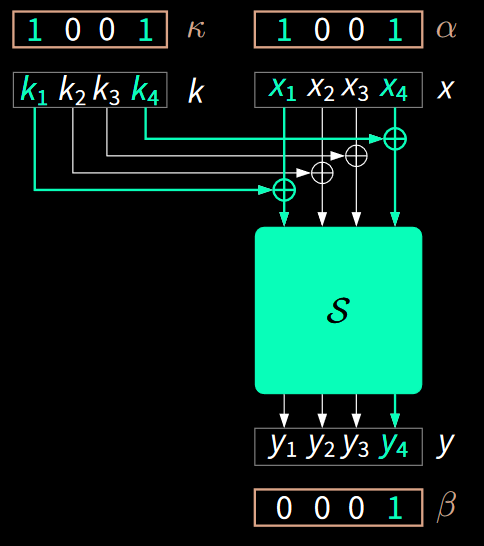For the masks in the image we have the following equations: $X_1 \oplus X_4 \oplus K_1 \oplus K_4 = Y_4 \\ X_1 \oplus X_4 \oplus Y_4 = K_1 \oplus K_4$

Given enough $(X, Y)$ pairs we get some information about the key.

In :
S = [12, 5, 6, 11, 9, 0, 10, 13, 3, 14, 15, 8, 4, 7, 1, 2]
lat = linear_approximation_table(S, 4)

#Investigate the lat
#print(np.matrix(lat))

In :
# Pick an entry that is not 0 -> 9-1 => 4 in the LAT
alpha = 0b1001 # 9
beta = 0b0001 # 1
kappa = alpha
print("LAT entry: ", lat[alpha][beta])

X = getrandbits(4)
print("X: ", bin(X)[2:].zfill(4))
K = getrandbits(4)
print("K: ", bin(K)[2:].zfill(4))
Y = S[X ^ K]
K1K4 = dot(X, alpha) ^ dot(Y, beta)

print(K1K4 == get_bit(K, 0, 4) ^ get_bit(K, 3, 4))

LAT entry:  4.0
X:  0000
K:  0101
False

In :
# Let's try more runs
t = 0
tries = 1000
for _ in range(tries):
X = getrandbits(4)
K = getrandbits(4)
Y = S[X ^ K]
K1K4 = dot(X, alpha) ^ dot(Y, beta)
t += K1K4 == get_bit(K, 0, 4) ^ get_bit(K, 3, 4) # Increment if true

print(t / tries) # clearly a bias and not random

0.753

In :
# Let's look at an entry equal to 0
alpha = 1
beta = 0
print("LAT entry: ", lat[alpha][beta])

t = 0
tries = 1000
for _ in range(tries):
X = getrandbits(4)
K = getrandbits(4)
Y = S[X ^ K]
K1K4 = dot(X, alpha) ^ dot(Y, beta)
t += K1K4 == get_bit(K, 0, 4) ^ get_bit(K, 3, 4) # Increment if true

print(t / tries) # close to .5 thus random

LAT entry:  0.0
0.488


Let's walk through another example. This one is taken from "Block cipher companion":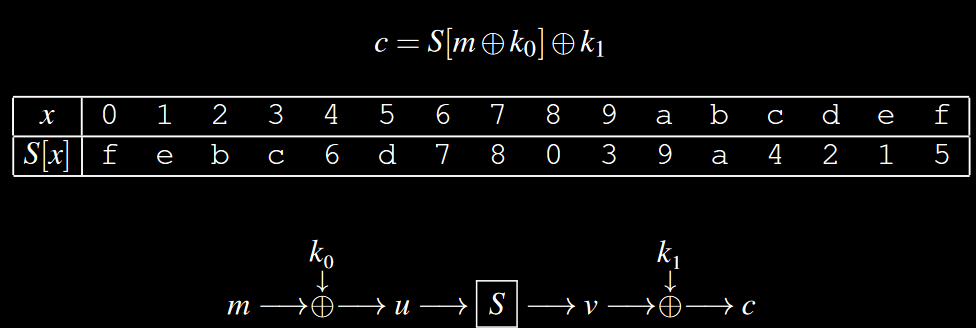Let

• $\alpha$ be the mask for $m$
• $\beta$ be the mask for $c$

If we play with the equations we get

• $\alpha \cdot m = \alpha \cdot k_0 \oplus \alpha \cdot u$ - always (1)
• $\alpha \cdot u = \beta \cdot v$ - with probability $p$ (2)
• $\beta \cdot v = \beta \cdot k_1 \oplus \beta \cdot c$ - always (3)

By equating (1) with (3) through (2)

• $\alpha \cdot m \oplus \alpha \cdot k_0 = \beta \cdot k_1 \oplus \beta \cdot c \iff \\ \alpha \cdot m \oplus \beta \cdot c = \alpha \cdot k_0 \oplus \beta \cdot k_1$
• On the left we have $m, c$ and on the right $k_0, k_1$
In :
S = [0xf, 0xe, 0xb, 0xc, 0x6, 0xd, 0x7, 0x8, 0x0, 0x3, 0x9, 0xa, 0x4, 0x2, 0x1, 0x5]
lat = linear_approximation_table(S, 4)

alpha = 0b1001
beta = 0b0010
print("LAT entry: ", lat[alpha][beta])

t = 0
tries = 1000
for _ in range(tries):
m = getrandbits(4)
k0 = getrandbits(4)
k1 = getrandbits(4)

u = m ^ k0
v = S[u]
c = v ^ k1

# lat[alpha][beta] = -6 => we xor with 1
t += dot(m, alpha) ^ dot(c, beta) ^ 1 == dot(k0, alpha) ^ dot(k1, beta)

print(t / tries) # clearly biased

LAT entry:  -6.0
0.864


Consider the following scenario: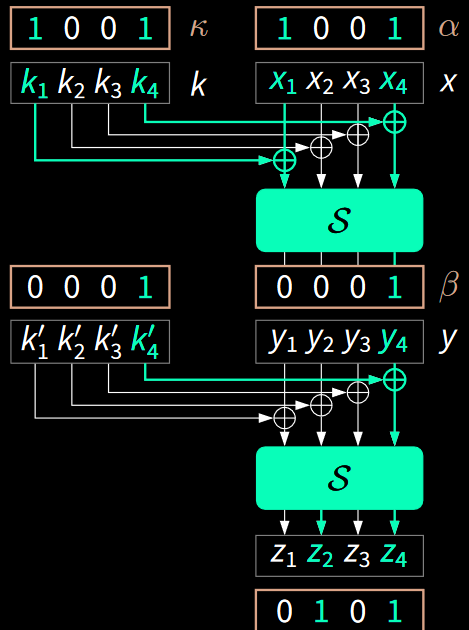$\alpha \cdot X \oplus \kappa \cdot K = \beta \cdot Y \\ \beta \cdot y \oplus \kappa' \cdot K' = \gamma \cdot z$

For this to work we need $\kappa' = \beta$. Otherwise we use other bits that we don't know anything about. Rewriting:

$\alpha \cdot X \oplus \kappa \cdot K = \beta \cdot Y \\ \beta \cdot y \oplus \beta \cdot K' = \gamma \cdot z$

Get some good masks from the table. Equating:

$X_1 \oplus X_4 \oplus K_1 \oplus K_4 \oplus K'_4 = Z_2 \oplus Z_4 \iff \\ X_1 \oplus X_4 \oplus Z_2 \oplus Z_4 = K_1 \oplus K_4 \oplus K'_4$

In :
S = [12, 5, 6, 11, 9, 0, 10, 13, 3, 14, 15, 8, 4, 7, 1, 2]
lat = linear_approximation_table(S, 4)

alpha = 0b1001
kappa = alpha
beta = 0b0001
gamma = 0b0101
print("LAT entry alpha beta: ", lat[alpha][beta])
print("LAT entry beta gamma: ", lat[beta][gamma])

t = 0
tries = 1000
for _ in range(tries):
X = getrandbits(4)
K = getrandbits(4)
K_ = getrandbits(4)

Y = S[X ^ K]
Z = S[Y ^ K_]

# lat[alpha][beta] = -6 => we xor with 1
t += dot(X, alpha) ^ dot(Z, gamma) == dot(K, alpha) ^ dot(K_, beta)

print(t / tries) # clearly biased

LAT entry alpha beta:  4.0
LAT entry beta gamma:  -4.0
0.372


### Pilling up lemma¶

The next question arises: If we know the bias of each Sbox approximation, what is the bias of the whole picture? How can we find it?

In :
# Consider the probabilities
p1 = count_matches(alpha, beta, S, 4) / (1<<4)
p2 = count_matches(beta, gamma, S, 4) / (1<<4)
print(p1, p2)

0.75 0.25


The approximation is correct if both are "wrong" or "correct". Assuming independence: $p = \underbrace{p_1 \cdot p_2}_{\text{correct}} + \underbrace{(1 - p_1) \cdot (1 - p_2)}_{\text{wrong}} \iff \\ p = \dfrac 1 2 + 2 \cdot \epsilon_1 \cdot \epsilon_2$

In :
p = p1 * p2 + (1 - p1) * (1 - p2)
print(p) # Notice that is around what we calculated above

0.375


The pilling up lemma

Let $X_i$ be independent boolean expressions with $p_i = P[X_i = 0] = \dfrac 1 2 + \epsilon_i$ we have the following probability: $$P[X_1 \oplus \dots \oplus X_n = 0] = \dfrac 1 2 + 2^{n-1}\prod_{i=1}^n \epsilon_i$$ Or in bias terms $$\epsilon_{1,...,n} = 2^{n-1}\prod_{i=1}^n \epsilon_i$$

Remark

• Lemma holds, in general, only when random variables are independent
In :
def pilling_up_lemma(eps_list):
n = len(eps_list)
return 2**(n-1) * np.prod(eps_list)

In :
eps1 = p1 - 1/2
eps2 = p2 - 1/2
print(eps1, eps2)

p_ = 1 / 2 + 2**1 * np.prod([eps1, eps2])
p_ # It's the same as above

0.25 -0.25

Out:
0.375

## Recovering the key¶

### Matsui 1¶

This algorithm will give 1 bit of information about the key per linear approximation. Assume we have $\alpha \cdot X \oplus \beta \cdot Y \oplus \kappa \cdot K = 0$ with a positive bias (this means the equation is correct most times)

Algorithm:

Input:

• Many Ciphertext / Plaintext pairs

Let

• $T_0, T_1$ - 2 counters

• For each pair $(M_i, C_i)$

• if $\alpha \cdot M_i \oplus \beta \cdot C_i = 0$ then $T_0++$
• if $\alpha \cdot M_i \oplus \beta \cdot C_i = 1$ then $T_1++$
• We learn the following

• If $T_0 > T_1 \Rightarrow \kappa \cdot K = 0$
• If $T_1 > T_0 \Rightarrow \kappa \cdot K = 1$

Cons

• Needs approx of all rounds
• Needs several approximations
• Learns only 1 bit of information. We need more equations to get more bits

Remark

• We need around $\dfrac 1 { \epsilon^2}$ messages
In :
def matsui1(M_list, C_list, alpha, beta):
T0, T1 = 0, 0
for m, c in zip(M_list, C_list):
if dot(m, alpha) ^ dot(c, beta) == 0:
T0+=1
else:
T1+=1
if T0 > T1:
return 0
else:
return 1

In :
# Going back to our first example
S = [12, 5, 6, 11, 9, 0, 10, 13, 3, 14, 15, 8, 4, 7, 1, 2]
lat = linear_approximation_table(S, 4)

K = getrandbits(4)
N = 1000

M_list = []
C_list = []
# Generate messages
for _ in range(N):
M = getrandbits(4)
C = S[M ^ K]
M_list.append(M)
C_list.append(C)

In :
alpha = 0b1001 # 9
beta = 0b0001 # 1
print("LAT entry: ", lat[alpha][beta])

LAT entry:  4.0

In :
K1K4 = dot(K, alpha)

In :
matsui1(M_list, C_list, alpha, beta) == K1K4 # True

Out:
True

Let's look at this example from "The block cipher companion":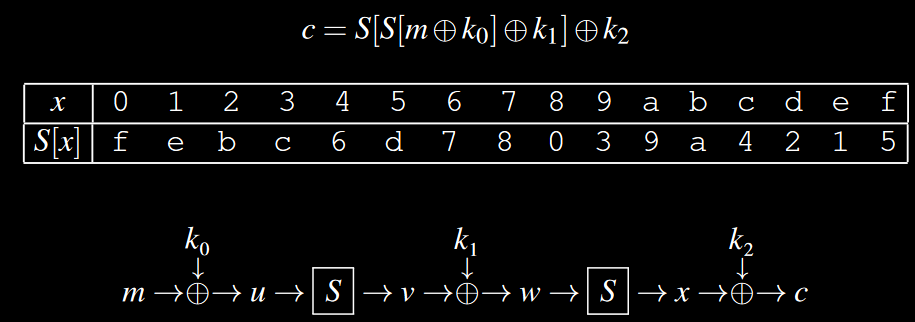In :
# Generate some ciphertext key pairs
S = [0xf, 0xe, 0xb, 0xc, 0x6, 0xd, 0x7, 0x8, 0x0, 0x3, 0x9, 0xa, 0x4, 0x2, 0x1, 0x5]
lat = linear_approximation_table(S, 4)

M_list, C_list = [], []
N = 100

k0 = getrandbits(4)
k1 = getrandbits(4)
k2 = getrandbits(4)

for _ in range(N):
m = getrandbits(4)
c = S[S[m ^ k0]^ k1]^ k2
M_list.append(m)
C_list.append(c)

In :
# alpha1 -> S -> alpha2 -> S -> alpha3

# looking at the LAT we see
print(lat[0xd][0xd]) # -6
# Therefore we can use
alpha1 = alpha2 = alpha3 = 0xd

# Attacking
print(matsui1(M_list, C_list, alpha1, alpha3) == dot(k0, alpha1) ^ dot(k1, alpha2) ^ dot(k2, alpha3))

-6.0
True


### Matsui 2¶

What the first algorithm didn't exploit was how strong the bias is (how different is from 0)

Assume we have an approximation to the first few rounds => we have a bias $\epsilon$ and a lot of message-ciphertext pairs.

• We can brute force more key bits and decrypt to the bias-known round => $C'$
• If $\alpha \cdot M = \beta \cdot C'$ increase $T_0$ else increase $T_1$
• The right key will behave similar to the bias.
• If we chose a large positive bias then $T_0 \gg T_1$ or reverse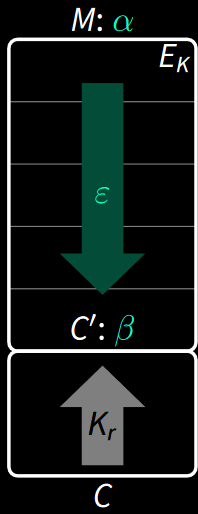Consider the following cipher from The block cipher companion: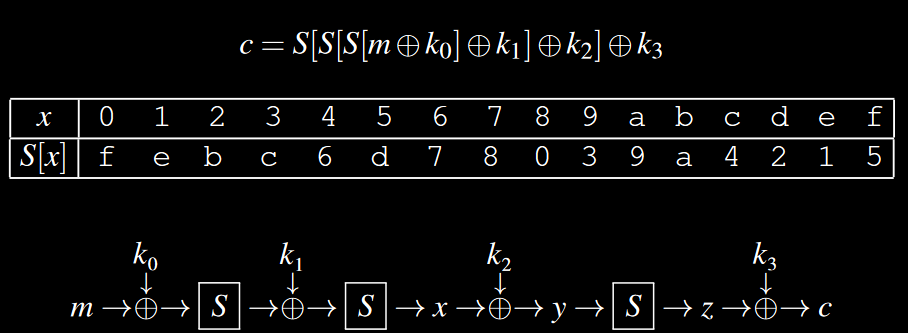In :
# Generate some ciphertext key pairs
S = [0xf, 0xe, 0xb, 0xc, 0x6, 0xd, 0x7, 0x8, 0x0, 0x3, 0x9, 0xa, 0x4, 0x2, 0x1, 0x5]
lat = linear_approximation_table(S, 4)

M_list, C_list = [], []
N = 1000

k0 = getrandbits(4)
k1 = getrandbits(4)
k2 = getrandbits(4)
k3 = getrandbits(4)

for _ in range(N):
m = getrandbits(4)
c = S[S[S[m ^ k0]^ k1]^ k2] ^ k3
M_list.append(m)
C_list.append(c)

In :
print(np.matrix(lat))

[[ 8.  0.  0.  0.  0.  0.  0.  0.  0.  0.  0.  0.  0.  0.  0.  0.]
[ 0. -2.  0.  2.  0. -2.  4. -2.  2.  4.  2.  0. -2.  0.  2.  0.]
[ 0.  2. -2.  0. -2.  0.  0.  2.  2.  4.  0.  2.  4. -2. -2.  0.]
[ 0.  4.  2.  2. -2.  2.  0.  0.  0.  0.  2. -2. -2. -2.  0.  4.]
[ 0.  0. -2.  2.  2. -2.  0.  0. -4.  0.  2.  2.  2.  2.  0.  4.]
[ 0. -2.  2.  0.  2.  4.  0.  2. -2.  4.  0. -2.  0.  2. -2.  0.]
[ 0. -2.  0.  2.  0.  2.  4.  2.  2. -4.  2.  0.  2.  0. -2.  0.]
[ 0.  0.  0.  0.  0.  4.  0. -4.  0.  0.  0.  0.  4.  0.  4.  0.]
[ 0.  0. -2.  2. -4.  0.  2.  2. -4.  0. -2. -2.  0.  0.  2. -2.]
[ 0. -2. -6.  0.  0.  2. -2.  0.  2.  0.  0. -2. -2.  0.  0.  2.]
[ 0. -2.  0. -6. -2.  0.  2.  0. -2.  0.  2.  0.  0. -2.  0.  2.]
[ 0.  0.  0.  0.  2. -2.  2. -2.  0.  0. -4. -4.  2. -2. -2.  2.]
[ 0.  0.  0.  0. -2. -2. -2. -2.  0.  0.  4. -4.  2.  2. -2. -2.]
[ 0. -2.  0.  2.  2.  0. -2.  0. -2.  0.  2.  0.  0. -6.  0. -2.]
[ 0.  2. -2.  0.  0.  2.  2. -4. -2.  0.  0.  2. -2.  0. -4. -2.]
[ 0. -4.  2.  2. -4.  0. -2. -2.  0.  0. -2.  2.  0.  0. -2.  2.]]

In :
# alpha1 - alpha 2 -> 1st Sbox
# alpha2 - alpha3 -> 2nd Sbox
# alpha3 - alpha4 - > 3rd Sbox

alpha1 = 0b1001 # 9
alpha2 = 0b0010 # 2
alpha3 = 0b1001 # 9
alpha4 = 0b0010 # 2

# or

alpha1 = alpha2 = alpha3 = alpha4 = 0xd

In :
eps1 = count_matches(alpha1, alpha2, S, 4) / (1<<4) - 1/2
eps2 = count_matches(alpha2, alpha3, S, 4) / (1<<4) - 1/2
eps3 = count_matches(alpha3, alpha4, S, 4) / (1<<4) - 1/2
print(eps1, eps2, eps3)

-0.375 -0.375 -0.375

In :
eps = pilling_up_lemma([eps1, eps2])
print(eps)
p = eps + 1/2
print(p) # Therefore we expect p correct cases

0.28125
0.78125

In :
1/eps**2

Out:
12.641975308641975
In :
def matsui2(M_list, C_list, alpha, beta, S_, key_bits = 4):
"""
Does R-1 key attack
Input:
M_list: {list} -- messages
C_list: {list} -- ciphertext
beta: {int} -- penultimate round mask
S_: {list} -- Sbox INVERSE
key_bits: {int}, default=4 -- number of key bits to search through

Return:
{list} -- list of differences. Take the max difference for the key
"""
t_diffs = []
for k in range(1<<key_bits): # Iterate through possible keys
t0, t1 = 0, 0
for m, c in zip(M_list, C_list):
# Reverse one round
c_ = c ^ k
c_ = S_[c_]
# Check if the statement is correct
if dot(m, alpha) == dot(c_, beta) :
t0+=1
else:
t1+=1
print(t0, t1)
t_diffs.append(t0 - t1)
return t_diffs

In :
k3

Out:
8
In :
S_ = inverse_sbox(S)
t_diffs = matsui2(M_list, C_list, alpha1, alpha3, S_)

123 877
748 252
249 751
755 245
773 227
112 888
769 231
239 761
888 112
227 773
761 239
231 769
252 748
877 123
245 755
751 249

In :
np.argmax(t_diffs)

Out:
8
In :
np.argsort(np.abs(t_diffs))[::-1]

Out:
array([ 8,  5, 13,  0,  9,  4, 11,  6, 10,  7, 14,  3, 15,  2, 12,  1],
dtype=int64)

## Bigger block cipher¶

Let's work on the SPN cipher we defined in the "Design of block ciphers" notebook

In [ ]:
# Substitute and permute

def substitute(x, sbox, l):
"""
Takes a 2**n bit number x and substitutes n-bit parts according to the n-bit sbox
Arguments
x: {int} --  input number of size 2**n bits
sbox: {Sbox} -- Sbox with input entries of size n bits
l: {int} -- Sbox input size in bits
Returns
{int} -- output sboxed
"""
word_size = 1 << l
y = 0
for i in range(0, word_size, l): # Steps of n-bits
y  = y << l # Shift 'l' positions to make space for l-bit sbox number
idx = (x >> (word_size - i - l)) & mask # Get n-bit index in the sbox
y = y | sbox[idx] # "append" the bit from x
return y

def permute(x, pbox, n):
"""
Takes a n-bit int x and permutes the bits from it according to the pbox
Arguments
x: {int}  -- n-bit int
pbox: {list} -- list of integers of length n
Return:
{int}
"""
y = 0
for p in pbox:
y = y << 1 # shift to make space for LSB
y = y ^ get_bit(x, p, n) # "append" the p'th bit from x
return y

class SPN:
def __init__(self, sbox, pbox, block_size = 16, sbox_input_size = 4):
self.sbox = sbox
self.sbox_ = inverse_sbox(sbox)
self.pbox = pbox
self.block_size = block_size
self.l = sbox_input_size

def key_schedule(self, k):
return [k >> i * 4 & 0xffff for i in range(4, -1, -1)]

def encrypt(self, m: int, k: int) -> int:
ks = self.key_schedule(k)

w = m
for i in range(len(ks) - 2):
u = w ^ ks[i]
v = substitute(u, self.sbox, self.l)
w = permute(v, self.pbox, self.block_size)

u = w ^ ks[-2]
v = substitute(u, S, self.l)
y = v ^ ks[-1]

return y

def decrypt(self, c: int, k: int) -> int:

ks = self.key_schedule(k)

v = ks[-1] ^ c
u = substitute(v, self.sbox_, self.l)
w = ks[-2] ^ u

for ki in ks[::-1][2:]:
v = permute(w, self.pbox, self.block_size)
u = substitute(v, self.sbox_, self.l)
w = u ^ ki
return w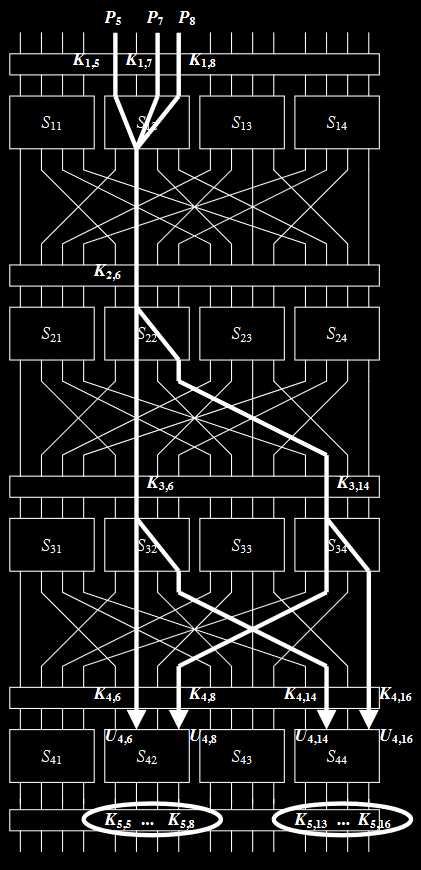In :
#Sbox and Pbox
S = [14, 4, 13, 1, 2, 15, 11, 8, 3, 10, 6, 12, 5, 9, 0, 7]
S_ = inverse_sbox(S)
P = [0, 4, 8, 12, 1, 5, 9, 13, 2, 6, 10, 14, 3, 7, 11, 15]

In :
lat = linear_approximation_table(S, 4)

In :
print(np.matrix(lat))

[[ 8.  0.  0.  0.  0.  0.  0.  0.  0.  0.  0.  0.  0.  0.  0.  0.]
[ 0.  0. -2. -2.  0.  0. -2.  6.  2.  2.  0.  0.  2.  2.  0.  0.]
[ 0.  0. -2. -2.  0.  0. -2. -2.  0.  0.  2.  2.  0.  0. -6.  2.]
[ 0.  0.  0.  0.  0.  0.  0.  0.  2. -6. -2. -2.  2.  2. -2. -2.]
[ 0.  2.  0. -2. -2. -4. -2.  0.  0. -2.  0.  2.  2. -4.  2.  0.]
[ 0. -2. -2.  0. -2.  0.  4.  2. -2.  0. -4.  2.  0. -2. -2.  0.]
[ 0.  2. -2.  4.  2.  0.  0.  2.  0. -2.  2.  4. -2.  0.  0. -2.]
[ 0. -2.  0.  2.  2. -4.  2.  0. -2.  0.  2.  0.  4.  2.  0.  2.]
[ 0.  0.  0.  0.  0.  0.  0.  0. -2.  2.  2. -2.  2. -2. -2. -6.]
[ 0.  0. -2. -2.  0.  0. -2. -2. -4.  0. -2.  2.  0.  4.  2. -2.]
[ 0.  4. -2.  2. -4.  0.  2. -2.  2.  2.  0.  0.  2.  2.  0.  0.]
[ 0.  4.  0. -4.  4.  0.  4.  0.  0.  0.  0.  0.  0.  0.  0.  0.]
[ 0. -2.  4. -2. -2.  0.  2.  0.  2.  0.  2.  4.  0.  2.  0. -2.]
[ 0.  2.  2.  0. -2.  4.  0.  2. -4. -2.  2.  0.  2.  0.  0.  2.]
[ 0.  2.  2.  0. -2. -4.  0.  2. -2.  0.  0. -2. -4.  2. -2.  0.]
[ 0. -2. -4. -2. -2.  0.  2.  0.  0. -2.  4. -2. -2.  0.  2.  0.]]

In :
alpha0 = 0b1011
alpha1 = 0b0100
alpha2 = 0b0101

In :
eps1 = count_matches(alpha0, alpha1, S, 4) / (1<<4) - 1/2
eps2 = count_matches(alpha1, alpha2, S, 4) / (1<<4) - 1/2
eps3 = count_matches(alpha1, alpha2, S, 4) / (1<<4) - 1/2
eps4 = count_matches(alpha1, alpha2, S, 4) / (1<<4) - 1/2
print(eps1, eps2, eps3, eps4)

0.25 -0.25 -0.25 -0.25

In :
eps = pilling_up_lemma([eps1, eps2, eps3, eps4])
print(eps)
print(1/eps**2)

-0.03125
1024.0

In :
def matsui2_big(M_list, C_list, alpha, beta, S_, key_bits = 8):
"""
Does R-1 key attack
Input:
M_list: {list} -- messages
C_list: {list} -- ciphertext
beta: {int} -- penultimate round mask
S_: {list} -- Sbox INVERSE
key_bits: {int}, default=4 -- number of key bits to search through

Return:
{list} -- list of differences. Take the max difference for the key
"""
t_diffs = []
for k in range(1<<key_bits): # Iterate through possible keys
k1, k2 = (k>>4) & 0xf, k & 0xf
t0, t1 = 0, 0
for m, c in zip(M_list, C_list):
# Reverse one round
c_ = c ^ (k1 << 8) ^ k2
c_ = substitute(c_, S_, 4)
# Check if the statement is correct
if dot(m, alpha) == dot(c_, beta):
t0+=1
else:
t1+=1
#print(t0, t1)
t_diffs.append(t0 - t1)
return t_diffs

In :
# Generate (m, c) pairs

spn = SPN(S, P)
K = getrandbits(48)
Ks = spn.key_schedule(K)

N = 10000
M_list = []
C_list = []
for _ in range(N):
m = getrandbits(16)
c = spn.encrypt(m, K)
M_list.append(m)
C_list.append(c)


In :
t_diffs = matsui2_big(M_list, C_list, 0x0b00, 0x0505, S_)

In :
(Ks[-1] & 0xf00) >> 8, Ks[-1] & 0xf

Out:
(10, 1)
In :
kk = np.argsort(np.abs(t_diffs))[::-1]

# Take first key
for key in kk[:1]:
print(key, (key & 0xf0) >> 4, key & 0xf)

161 10 1


## Defense against linear crypntalysis¶

In [ ]: